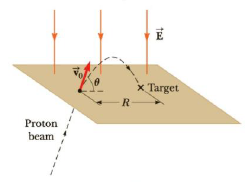Chapter 15, Problem 70AP

Chapter
Section
Textbook Problem

Protons are projected with an initial speed v0 = 9 550 m/s into a region where a uniform electric field of magnitude E = 720 N/C is present (Fig. P15.70). The protons are to hit a target that lies a horizontal distance of 1.27 mm from the point where the protons are launched. Find (a) the two projection angles θ that will result in a hit and (b) the total duration of flight for each of the two trajectories.Figure P15.70

(a)

To determine

The two projection angles.

Explanation

Given info: The electric field (E) is 720 N/C. The initial speed (v0) is 9550 m/s. Total horizontal distance (R) is 1.27 mm.

Formula to calculate the maximum horizontal range is,

R=v02sin2θay

• θ is the projection angle.
• ay is the vertical acceleration.

The vertical acceleration is,

|ay|=eEm

• m is the mass of proton.

Therefore,

R=mv02sin2θeE

On Re-arranging,

θ=12sin1(eERmv02)

Substitute 1.67×1027kg for m, 1.27 mm for R, 720 N/C for E, 9550 m/s for v0 and 1

(b)

To determine

The total duration of flight.

Still sussing out bartleby?

Check out a sample textbook solution.

See a sample solution

The Solution to Your Study Problems

Bartleby provides explanations to thousands of textbook problems written by our experts, many with advanced degrees!

Get Started